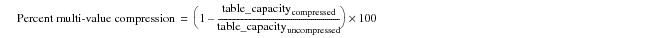# 15.00 - Calculating Multi-Value Percent Compression - Teradata Database

prodname
vrm_release
15.00
category
User Guide
featnum
B035-1094-015K

### Calculating Multi-Value Percent Compression

Percent compression is a normalized measure of the increase in total table capacity achieved by multi-value compression. “Equation 2: Percent Multi-Value Compression” is the equation for computing percent compression.

#### Equation 2: Percent Multi-Value Compressionwhere:

 This term … Specifies … table_capacitycompressed the value calculated by “Equation 4: Table Capacity for Multi-Value Compression” on page 726. table_capacityuncompressed the value calculated by “Equation 3: Uncompressed Table Capacity” on page 725.